# Conditions for Equilibrium in 2D Notes | Study Engineering Mechanics - Mechanical Engineering

## Mechanical Engineering: Conditions for Equilibrium in 2D Notes | Study Engineering Mechanics - Mechanical Engineering

The document Conditions for Equilibrium in 2D Notes | Study Engineering Mechanics - Mechanical Engineering is a part of the Mechanical Engineering Course Engineering Mechanics.
All you need of Mechanical Engineering at this link: Mechanical Engineering

Equilibrium as the condition in which the resultant of all forces and moments acting on a body is zero. Stated in another way, a body is in equilibrium if all forces and moments applied to it are in balance. These requirements are contained in the vector equations of equilibrium, Eqs. 3/1, which in two dimensions may be written in scalar form as

ΣFx = 0             Σ Fy = 0 ΣMo = 0      (3/2)

The third equation represents the zero sum of the moments of all forces about any point O on or off the body. Equations 3/2 are the necessary and sufﬁcient conditions for complete equilibrium in two dimensions. They are necessary conditions because, if they are not satisﬁed, there can be no force or moment balance. They are sufﬁcient because once they are satisﬁed, there can be no imbalance, and equilibrium is assured. These equations show that the acceleration of the mass center of a body is proportional to the resultant force ΣF acting on the body. Consequently, if a body moves with constant velocity (zero acceleration), the resultant force on it must be zero, and the body may be treated as in a state of translational equilibrium. For complete equilibrium in two dimensions, all three of Eqs. 3/2 must hold. However, these conditions are independent requirements, and one may hold without another. Take, for example, a body which slides along a horizontal surface with increasing velocity under the action of applied forces. The force–equilibrium equations will be satisﬁed in the vertical direction where the acceleration is zero, but not in the horizontal direction. Also, a body, such as a ﬂywheel, which rotates about its ﬁxed mass center with increasing angular speed is not in rotational equilibrium, but the two force–equilibrium equations will be satisﬁed.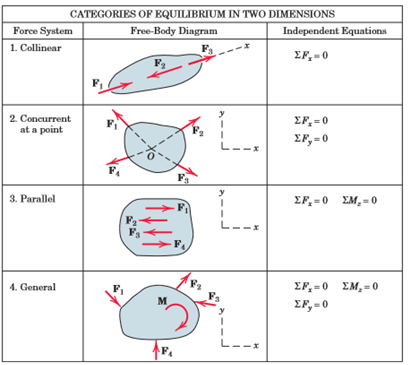In addition to Eqs. 3/2, there are two other ways to express the general conditions for the equilibrium of forces in two dimensions. The ﬁrst way is illustrated in Fig. 3/6, parts (a) and (b). For the body shown in Fig. 3/6a, if ΣMA = 0, then the resultant, if it still exists, cannot be a couple, but must be a force R passing through A. If now the equation ΣFx = 0 holds, where the x-direction is arbitrary, it follows from Fig. 3/6b that the resultant force R, if it still exists, not only must pass through A, but also must be perpendicular to the x-direction as shown. Now, if ΣMB = 0, where B is any point such that the line AB is not perpendicular to the x-direction, we see that R must be zero, and thus the body is in equilibrium. Therefore, an alternative set of equilibrium equations is

ΣFx = 0  ΣMA = 0     ΣMB = 0

where the two points A and B must not lie on a line perpendicular to the x-direction. A third formulation of the equilibrium conditions may be made for a coplanar force system. This is illustrated in Fig. 3/6, parts (c) and (d). Again, if ΣMA = 0 for any body such as that shown in Fig. 3/6c, the resultant, if any, must be a force R through A. In addition, if ΣMB 0, the resultant, if one still exists, must pass through B as shown in Fig. 3/6d. Such a force cannot exist, however, if ΣMC = 0, where C is not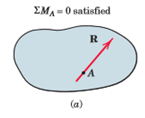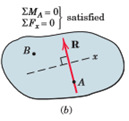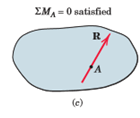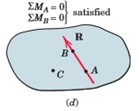collinear with A and B. Thus, we may write the equations of equilibrium as

ΣMA = 0     ΣMB = 0    ΣMC = 0

where A, B, and C are any three points not on the same straight line. When equilibrium equations are written which are not independent, redundant information is obtained, and a correct solution of the equations will yield 0

0. For example, for a general problem in two dimensions with three unknowns, three moment equations written about three points which lie on the same straight line are not independent. Such equations will contain duplicated information, and solution of two of them can at best determine two of the unknowns, with the third equation merely verifying the identity 0 = 0.

The document Conditions for Equilibrium in 2D Notes | Study Engineering Mechanics - Mechanical Engineering is a part of the Mechanical Engineering Course Engineering Mechanics.
All you need of Mechanical Engineering at this link: Mechanical EngineeringUse Code STAYHOME200 and get INR 200 additional OFF

## Engineering Mechanics

25 videos|71 docs|39 tests

Track your progress, build streaks, highlight & save important lessons and more!

,

,

,

,

,

,

,

,

,

,

,

,

,

,

,

,

,

,

,

,

,

;